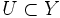# Quotient map

## Definition

### Symbol-free definition

A continuous map between topological spaces is termed a quotient map if it is surjective, and if a set in the range space is open iff its inverse image is open in the domain space.

### Definition with symbols

Let$X,Y$ be topological spaces and$f:X \to Y$ be continuous maps.$f$ is termed a quotient map if it is sujective and if$U \subset Y$ is open iff$f^{-1}(U)$ is open in$X$.

## Significance

Given a topological space$X$, a set$Y$ and a surjective map$f:X \to Y$, we can prescribe a unique topology on$Y$, the so-called quotient topology, such that$f$ is a quotient map. Moreover, this is the coarsest topology for which$f$ becomes continuous.

Also, the study of a quotient map is equivalent to the study of the equivalence relation on$X$ given by$x \simeq y \iff f(x) = f(y)$.